# Section 4 5 Graphs of Sine and Cosine

• Slides: 13
Download presentation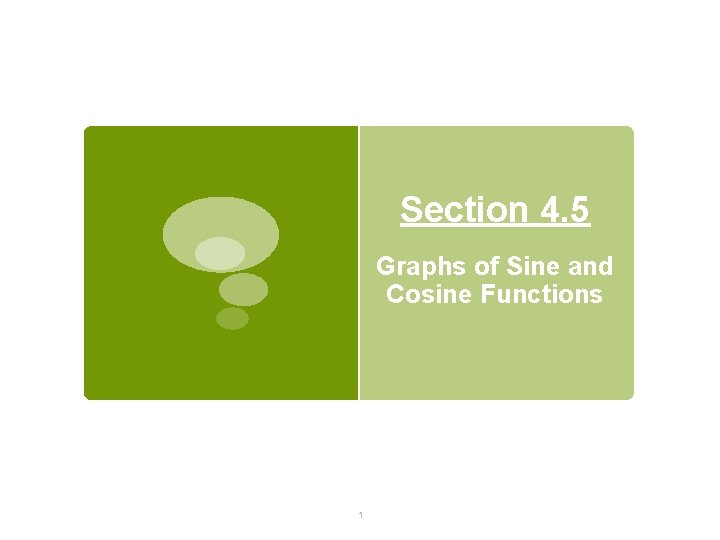Section 4. 5 Graphs of Sine and Cosine Functions 1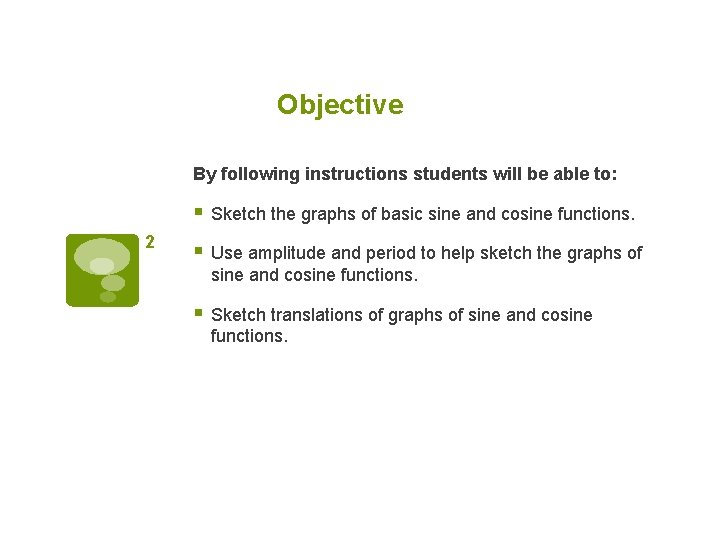Objective By following instructions students will be able to: § Sketch the graphs of basic sine and cosine functions. 2 § Use amplitude and period to help sketch the graphs of sine and cosine functions. § Sketch translations of graphs of sine and cosine functions.Sine Graph y=asin(bx-c)+d 3 intercepts maximum minimum period = one cycle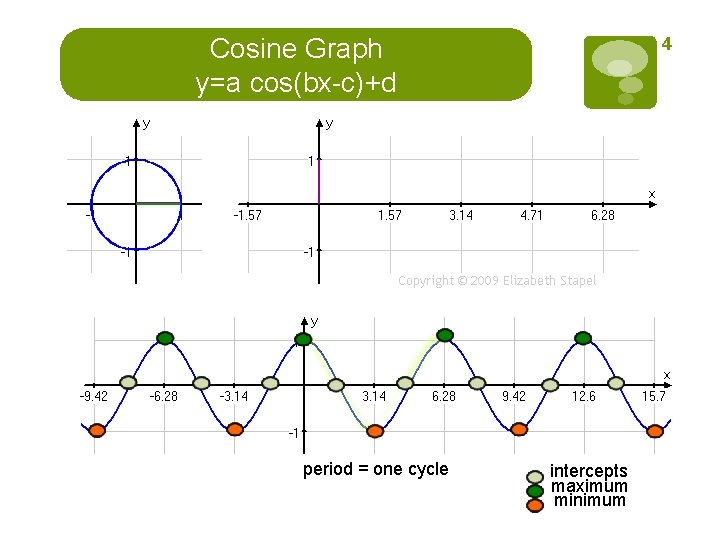Cosine Graph y=a cos(bx-c)+d period = one cycle 4 intercepts maximum minimumAmplitude and Period of Sine and Cosine Curves Amplitude = |a| 5 If a>1 , vertical stretch. If -1<a<1, vertical shrink. The range of the amplitude is Period Left and Right Endpoints Vertical Translation Left Endpoint Right Endpoint If d>1, graph shifts d units up. If d<1, shifts d units down. .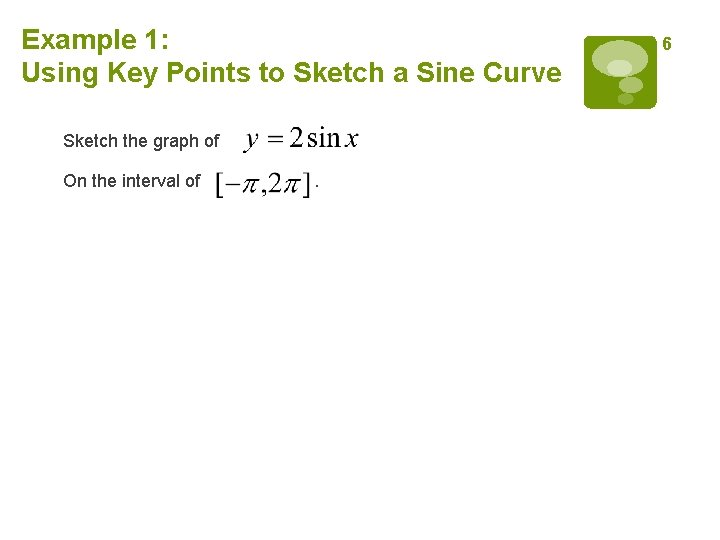Example 1: Using Key Points to Sketch a Sine Curve Sketch the graph of On the interval of . 6Example 2: Vertical Shrinking and Stretching On the same coordinate axes, sketch the graph of each function. a) b) c) 7Example 3: Horizontal Stretching Sketch the graph of . 89 Example 4: Horizontal Translation Analyze the graph of .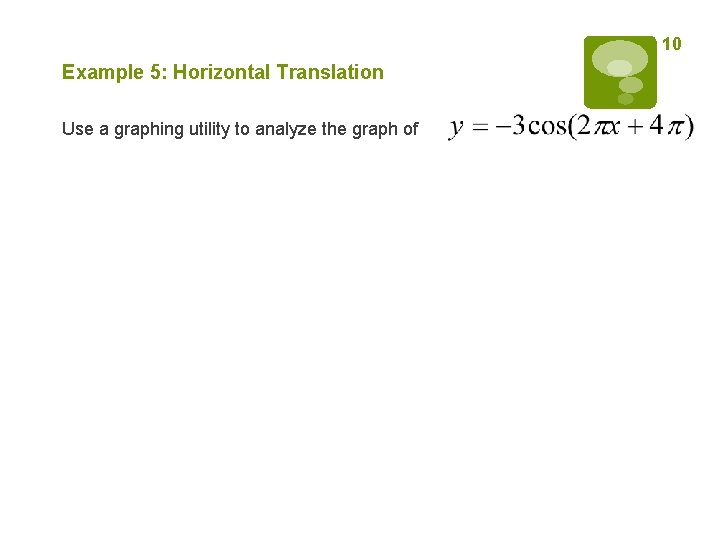10 Example 5: Horizontal Translation Use a graphing utility to analyze the graph of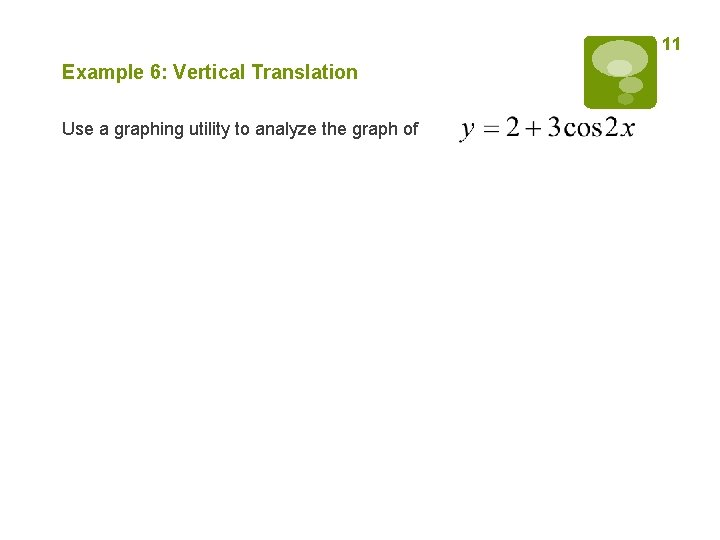11 Example 6: Vertical Translation Use a graphing utility to analyze the graph of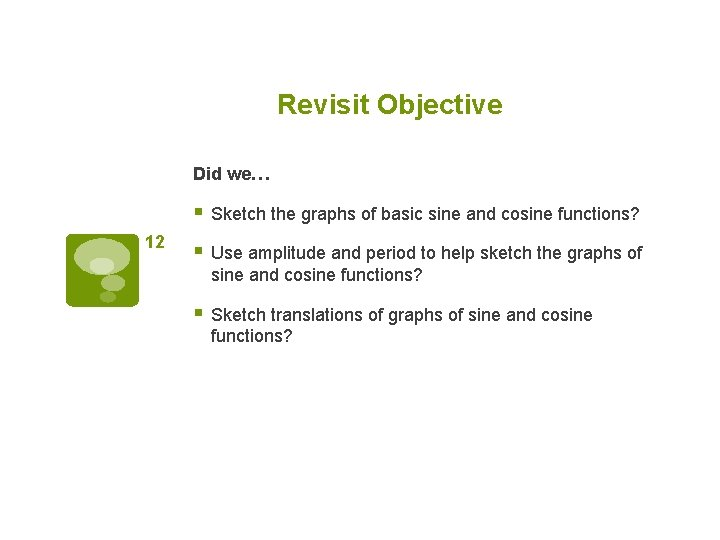Revisit Objective Did we… § Sketch the graphs of basic sine and cosine functions? 12 § Use amplitude and period to help sketch the graphs of sine and cosine functions? § Sketch translations of graphs of sine and cosine functions?Homework § Pg 330 #1 -65 ODD 13